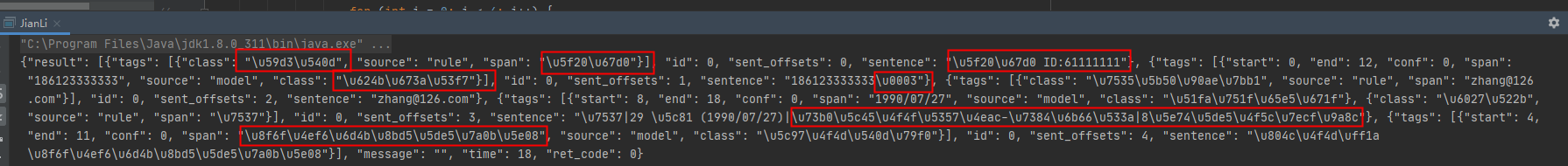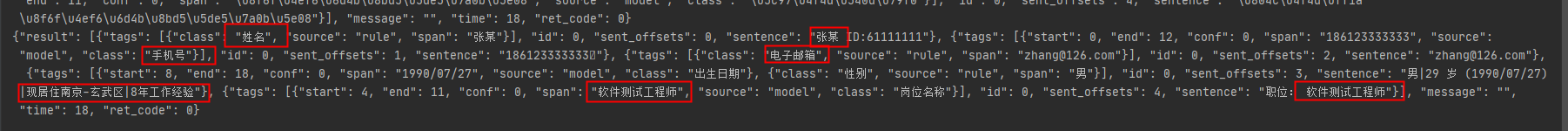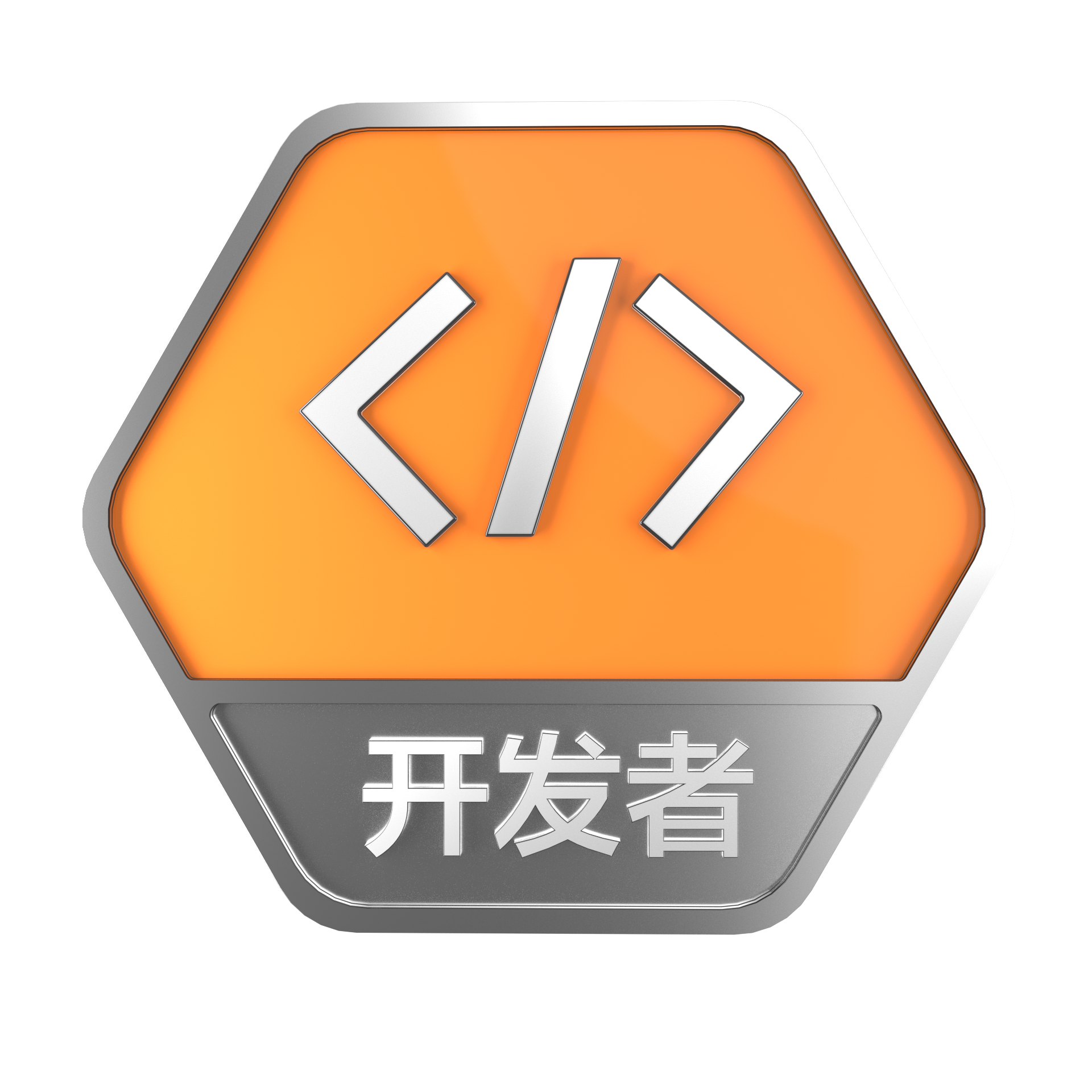响应返回JSON数据时出现的unicode编码问题

+关注继续查看

问题描述解决策略

public static String decodeUnicode(String theString) {
char aChar;
int len = theString.length();
StringBuffer outBuffer = new StringBuffer(len);
for (int x = 0; x < len;) {
aChar = theString.charAt(x++);
if (aChar == '\\') {
aChar = theString.charAt(x++);
if (aChar == 'u') {
int value = 0;
for (int i = 0; i < 4; i++) {
aChar = theString.charAt(x++);
switch (aChar) {
case '0':
case '1':
case '2':
case '3':
case '4':
case '5':
case '6':
case '7':
case '8':
case '9':
value = (value << 4) + aChar - '0';
break;
case 'a':
case 'b':
case 'c':
case 'd':
case 'e':
case 'f':
value = (value << 4) + 10 + aChar - 'a';
break;
case 'A':
case 'B':
case 'C':
case 'D':
case 'E':
case 'F':
value = (value << 4) + 10 + aChar - 'A';
break;
default:
throw new IllegalArgumentException("Malformed   \\uxxxx   encoding.");
}
}
outBuffer.append((char) value);
} else {
if (aChar == 't')
aChar = '\t';
else if (aChar == 'r')
aChar = '\r';
else if (aChar == 'n')
aChar = '\n';
else if (aChar == 'f')
aChar = '\f';
outBuffer.append(aChar);
}
} else
outBuffer.append(aChar);
}
return outBuffer.toString();
}

测试用例

//响应回的代码是对象类型。
ServiceResponse response = client.getResponse(request);
//但是响应体对象中有我们需要的String_json时我们要将他获取出来，比如
String strRes=response.getString;
//将此str放入传参中调用方法

String s1=decodeUnicode(strRes);

String s1=decodeUnicode(response.getString);

测试结果参考链接：

json中包含Unicode转码问题ajax请求后台，返回json格式数据，模板！

920 0MySQL---数据库从入门走向大神系列(三)-修改数据库编码/DOS窗口编码

1224 0Code Like a Pythonista: Idiomatic Python David Goodger goodger@python.org http://python.net/~goodger In this interactive tutorial, we'll cover man...
1035 01116 0iPhone开发入门守则：Objective-C编码规范--系列教程
823 0757 0vim 创建Python脚本时候自动补全解释器和编码方法
763 01600 0vim 创建Python脚本时候自动补全解释器和编码方法
907 0[算法系列之十六]数据压缩之游程编码
1817 0+关注
28

0

+ 订阅

《Nacos架构&原理》

《看见新力量：二》电子书